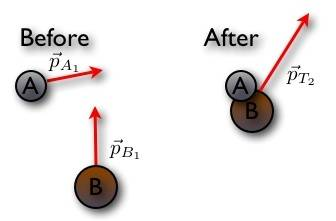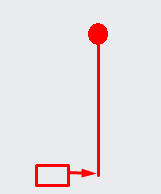# When is Energy/Momentum/Angular Momentum really conserved?

## Homework Statement

So during collisions between 2 point masses, I know that momentum is always conserved, but energy may or may not be conserved.Now, in this case, where we have a block colliding with a pivoted rod, I know that angular momentum is always conserved, but neither energy or momentum is.Now, in the last case, if I get rid of the pivot, I believe that energy, momentum, AND angular momentum are conserved.Can somebody explain the conditions for which energy, momentum, and angular momentum is conserved?

## Homework Equations

$KE_i = KE_f$
$p_i = p_f$
$L_i = L_f$

## The Attempt at a Solution

[/B]
From my understanding, it's if there are no "external forces" for momentum and angular momentum. I'm not sure about energy. However, for the 2nd case, what "external force" is there? I understand there's some force from the pivot point on the rod, but why would that be "external"? And if that were considered external, why wouldn't the force from the block on the rod be considered as "external" in the 3rd case?

Thanks!

gneill
Mentor
From my understanding, it's if there are no "external forces" for momentum and angular momentum. I'm not sure about energy.
Momentum is always conserved unless there are external forces acting.
Mechanical energy can change in an isolated system if that system includes non-mechanical energy storage mechanisms (such as energy stored as heat or in chemical substances) or can be lost to heat due to friction or locked away in mechanically deformed materials (perhaps initially as heat which is then radiated away).
However, for the 2nd case, what "external force" is there? I understand there's some force from the pivot point on the rod, but why would that be "external"?
What holds the pin in place? Can you draw a surface around your system (like a Gaussian surface) that includes the pin and everything that supports it?
And if that were considered external, why wouldn't the force from the block on the rod be considered as "external" in the 3rd case?
Because the block is part of your system: You're looking at the block and rod as the system.

By the way, energy need not be conserved if the collision is not perfectly elastic. If the block sticks to the rod or if there is a non-unity coefficient of restitution for the materials there will be energy loss.

haruspex
•